Next: Rotational flattening Up: Rotating reference frames Previous: Centrifugal acceleration

# Coriolis force

We have now accounted for the first fictitious force,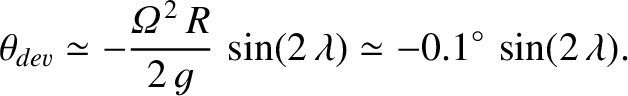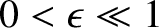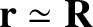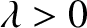, appearing in Equation (6.9). Let us now investigate the second, which takes the form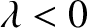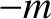, and is called the Coriolis force. Obviously, this force only affects objects that are moving in the rotating reference frame.

Consider a particle of massfree-falling under gravity in our rotating reference frame. As before, we define Cartesian axes in the rotating frame such that the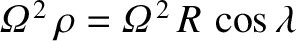-axis points vertically upward and the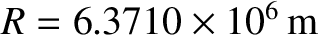- and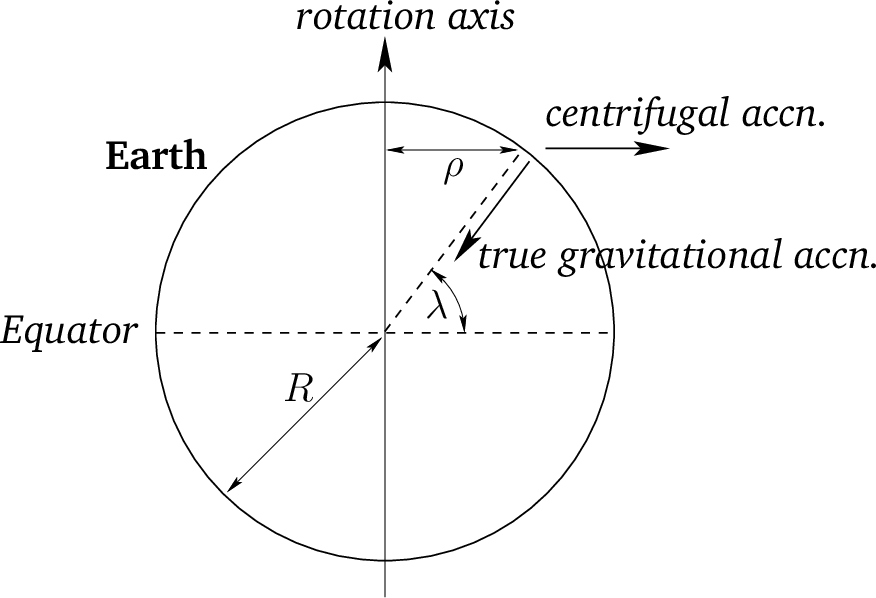-axes are horizontal, with the-axis pointing directly northward and the-axis pointing directly westward. It follows, from Equation (6.9), that the Cartesian equations of motion of the particle in the rotating reference frame take the form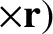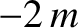(6.19)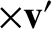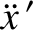(6.20) and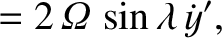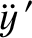(6.21)

Here,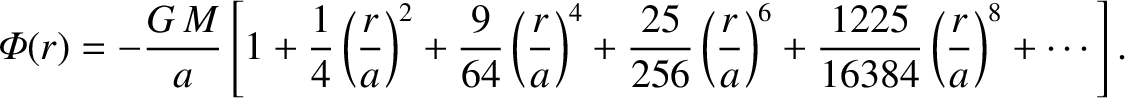is the local acceleration due to gravity. In the preceding three equations, we have neglected the centrifugal acceleration, for the sake of simplicity. This is reasonable, because the only effect of the centrifugal acceleration is to slightly modify the magnitude and direction of the local gravitational acceleration. We have also neglected air resistance, which is less reasonable.

Consider a particle that is dropped (at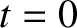) from rest a height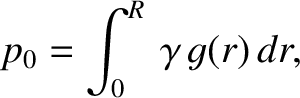above the Earth's surface. The following solution method exploits the fact that the Coriolis force is much smaller in magnitude that the force of gravity. Hence,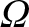can be treated as a small parameter. To lowest order (i.e., neglecting), the particle's vertical motion satisfies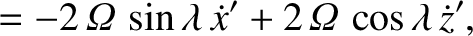, which can be solved, subject to the initial conditions, to give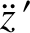(6.22)

Substituting this expression into Equations (6.19) and (6.20), neglecting terms involving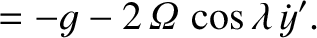, and solving subject to the initial conditions, we obtain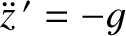and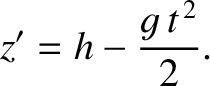(6.23)

In other words, the particle is deflected eastward (i.e., in the negative-direction). The particle hits the ground when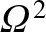. Hence, the net eastward deflection of the particle as it strikes the ground is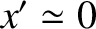(6.24)

This deflection is in the same direction as the Earth's rotation (i.e., west to east) and is greatest at the equator, and zero at the poles. A particle dropped from a height of 100m at the equator is deflected by about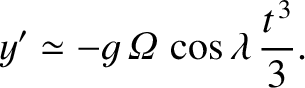.

Consider a particle launched horizontally with some fairly large velocity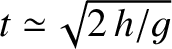(6.25)

Here,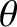is the compass bearing of the velocity vector (so north is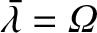, east is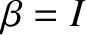, etc.). Neglecting any vertical motion, Equations (6.19) and (6.20) yield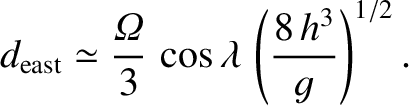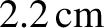(6.26) and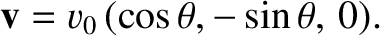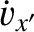(6.27)

which can be integrated to give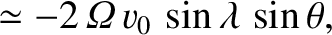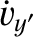(6.28) and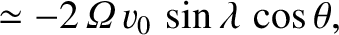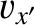(6.29)

To lowest order in, the preceding equations are equivalent to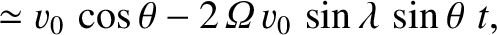(6.30) and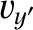(6.31)

It follows that the Coriolis force causes the compass bearing of the particle's velocity vector to rotate steadily as time progresses. The rotation rate is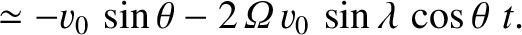(6.32)

Hence, the rotation is clockwise (if we look from above) in the northern hemisphere, and counterclockwise in the southern hemisphere. The rotation rate is zero at the equator and greatest at the poles.

The Coriolis force has a significant effect on terrestrial weather patterns. Near equatorial regions, the Sun's intense heating of the Earth's surface causes hot air to rise. In the northern hemisphere, this causes cooler air to move in a southerly direction toward the equator. The Coriolis force deflects this moving air in a clockwise sense (if we look from above), resulting in the trade winds, which blow toward the southwest. In the southern hemisphere, the cooler air moves northward and is deflected by the Coriolis force in a counterclockwise sense, resulting in trade winds that blow toward the northwest. Furthermore, as air flows from high- to low-pressure regions, the Coriolis force deflects the air in a clockwise/counterclockwise manner in the northern/southern hemisphere, producing cyclonic rotation. (See Figure 6.3.) It follows that cyclonic rotation is counterclockwise (seen from above) in the northern hemisphere, and clockwise in the southern hemisphere. Thus, this is the direction of rotation of tropical storms (e.g., hurricanes, typhoons) in each hemisphere.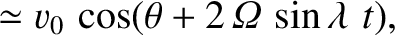Next: Rotational flattening Up: Rotating reference frames Previous: Centrifugal acceleration
Richard Fitzpatrick 2016-03-31